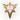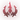## 符文系

•精密（强化攻击和持续伤害）
•主宰（爆发伤害并前往目标）
•巫术（强化技能和资源控制）
•坚决（耐久和控制）
•启迪（创造性的工具并扭转常规）

### 属性碎片

Option 1 Option 2 Option 3
Slot 1
Offense5.4 bonus Attack Damage or 9 Ability Power (Adaptive)10% bonus attack speed1% − 10% (based on level) cooldown reduction
Slot 2
Flex5.4 bonus Attack Damage or 9 Ability Power (Adaptive)6 bonus armor8 bonus magic resistance
Slot 3
Defense15 − 90 (based on level) bonus health6 bonus armor8 bonus magic resistance

### 转换

When the game starts the chat informs of these substitutions.

## 符文页

• 1-9级的玩家无法新建或编辑符文页。
• 10级开始可以新建和编辑符文页。
• 每个新账号有2个空白符文页。可以在商城花费或购买额外的符文页。
• S8季前赛阶段符文系统重铸，玩家在重铸前拥有的符文页会转为新的符文页，并会收到额外的补偿。
• 每个账号最多有25个符文页（不包括预设符文页）。
• 你可以选择隐藏预设符文页。

## Trivia

• During development, Precision and Sorcery were originally going to be Primary path only.
• Runes and their surrounding areas have distinct visual appearances which bear similarities to certain Runeterran factions. This may be intentional in order to reflect the location of the World Runes:
•Precision - Golden cityscape of
•Domination - fortification
•Sorcery - Mountains of
•Resolve - Riverlands of Southern
•Inspiration - Beneath the heavens atop
• The following statements generalize the changes made to compensate the loss of AD from Marks and Health/Armor from Seals, which were almost unanimously used due to the lack of options (and thus the game has been balanced, for example, around most champions having +9 Armor from Seals). The exact values may vary for specific cases, as Riot also took this opportunity to better distinguish classes (e.g. tanks from non-tanks; ranged from melee), as well as buff/nerf specific champions.
• AD champions had the base damage on their abilities increased by 5.
• Most champions had their increased by 9.
• Most mages had their increased by 12 (+12), instead. Primarily excepting junglers.
• More runes and paths may be added in the game.
• In Year in Review 2017 a joke phrase was added to each champion. A list with all phrases can be found over here.
• The number of all rune combinations can be seen below.
Calculation of rune combinations
Count Paths Calculation
Primary path Secondary path Primary path Secondary path Total Combinations
1PrecisionDomination 4*3*3*3=108 3*3+3*4+4*3=33 108*33=3564
1PrecisionSorcery 4*3*3*3=108 3*3+3*3+3*3=27 108*27=2916
1PrecisionResolve 4*3*3*3=108 3*3+3*3+3*3=27 108*27=2916
1PrecisionInspiration 4*3*3*3=108 3*3+3*3+3*3=27 108*27=2916
1DominationSorcery 4*3*3*4=144 3*3+3*3+3*3=27 144*27=3888
1DominationResolve 4*3*3*4=144 3*3+3*3+3*3=27 144*27=3888
1DominationInspiration 4*3*3*4=144 3*3+3*3+3*3=27 144*27=3888
1SorceryResolve 3*3*3*3=81 3*3+3*3+3*3=27 81*27=2187
1SorceryInspiration 3*3*3*3=81 3*3+3*3+3*3=27 81*27=2187
1ResolveInspiration 3*3*3*3=81 3*3+3*3+3*3=27 81*27=2187
1DominationPrecision 4*3*3*4=144 3*3+3*3+3*3=27 144*27=3888
1SorceryPrecision 3*3*3*3=81 3*3+3*3+3*3=27 81*27=2187
1ResolvePrecision 3*3*3*3=81 3*3+3*3+3*3=27 81*27=2187
1InspirationPrecision 3*3*3*3=81 3*3+3*3+3*3=27 81*27=2187
1SorceryDomination 3*3*3*3=81 3*3+3*4+4*3=33 81*33=2673
1ResolveDomination 3*3*3*3=81 3*3+3*4+4*3=33 81*33=2673
1InspirationDomination 3*3*3*3=81 3*3+3*4+4*3=33 81*33=2673
1ResolveSorcery 3*3*3*3=81 3*3+3*3+3*3=27 81*27=2187
1InspirationSorcery 3*3*3*3=81 3*3+3*3+3*3=27 81*27=2187
1InspirationResolve 3*3*3*3=81 3*3+3*3+3*3=27 81*27=2187
Total

• Prior to the introduction of Shards, players received a bonus based on the combination of their sets (e.g. Sorcery + Precision). These combinations had specific Titles, which are shared with the corresponding presets. The following is listed for archive purposes:
Secondary ► Precision Domination Sorcery Resolve Inspiration
Primary ▼
Precision The Merciless Elite The Brazen Prefect The Eternal Champion The Savant
Domination The Twisted Surgeon The Aether Blade The Immortal Butcher The Wicked Maestro
Sorcery The Incontestable Spellslinger The Calamity The Ancient One The Cryptic
Resolve The Imperious Behemoth The Leviathan The Arcane Colossus The Enlightened Titan
Inspiration The Elegant Duelist The Ruthless Visionary The Stargazer The Timeless
• 'The Perfect' preset was originally called 'The Prefect', as with the Set Title. It is unknown why this was changed.

## Patch History

;V9.23
V9.2
• Slot 1 and 2 adaptive shard reduced to (5.4 bonus AD or 9 AP) from (6 bonus AD or 10 AP).
• Slot 2 and 3 armor shard increased to 6 bonus armor from 5.
• Slot 2 and 3 magic resistance shard increased to 8 bonus magic resistance from 6.
V9.1
• Offense attack speed shard increased to 10% bonus attack speed from 9%.
V8.23
V8.11
V8.6
V8.4
V7.23
• Added an option to hide preset pages.